# Interpretable Machine LearningInterpretable Machine Learning - Extracting human understandable insights from any Machine Learning model. A Guide for Making Black Box Models Explainable. Machine Learning doesn’t have to be a black box anymore. What use is a good model if we cannot explain the results to others. Interpretability is as important as creating a model.

In his book ‘Interpretable Machine Learning’, Christoph Molnar beautifully encapsulates the essence of ML interpretability through this example: Imagine you are a Data Scientist and in your free time you try to predict where your friends will go on vacation in the summer based on their facebook and twitter data you have. Now, if the predictions turn out to be accurate, your friends might be impressed and could consider you to be a magician who could see the future. If the predictions are wrong, it would still bring no harm to anyone except to your reputation of being a “Data Scientist”. Now let’s say it wasn’t a fun project and there were investments involved. Say, you wanted to invest in properties where your friends were likely to holiday. What would happen if the model’s predictions went awry?You would lose money. As long as the model is having no significant impact, it’s interpretability doesn’t matter so much but when there are implications involved based on a model’s prediction, be it financial or social, interpretability becomes relevant.

## Explainable Machine Learning

Interpret means to explain or to present in understandable terms. In the context of ML systems, interpretability is the ability to explain or to present in understandable terms to a human[Finale Doshi-Velez] Machine Learning models have been branded as ‘Black Boxes’ by many. This means that though we can get accurate predictions from them, we cannot clearly explain or identify the logic behind these predictions. But how do we go about extracting important insights from the models? What things are to be kept in mind and what features or tools will we need to achieve that? These are the important questions which come to mind when the issue of Model Explainability is raised.

### Importance of interpretability

The question that some of the people often ask is why aren’t we just content with the results of the model and why are we so hell-bent on knowing why a particular decision was made? A lot of this has to do with the impact that a model might have in the real world. For models which are merely meant to recommend movies will have a far less impact than the ones created to predict the outcome of a drug.

Here is a big picture of explainable machine learning. In a way, we capture the world by collecting raw data and use that data to make further predictions. Essentially, Interpretability is just another layer on the model that helps humans to understand the process.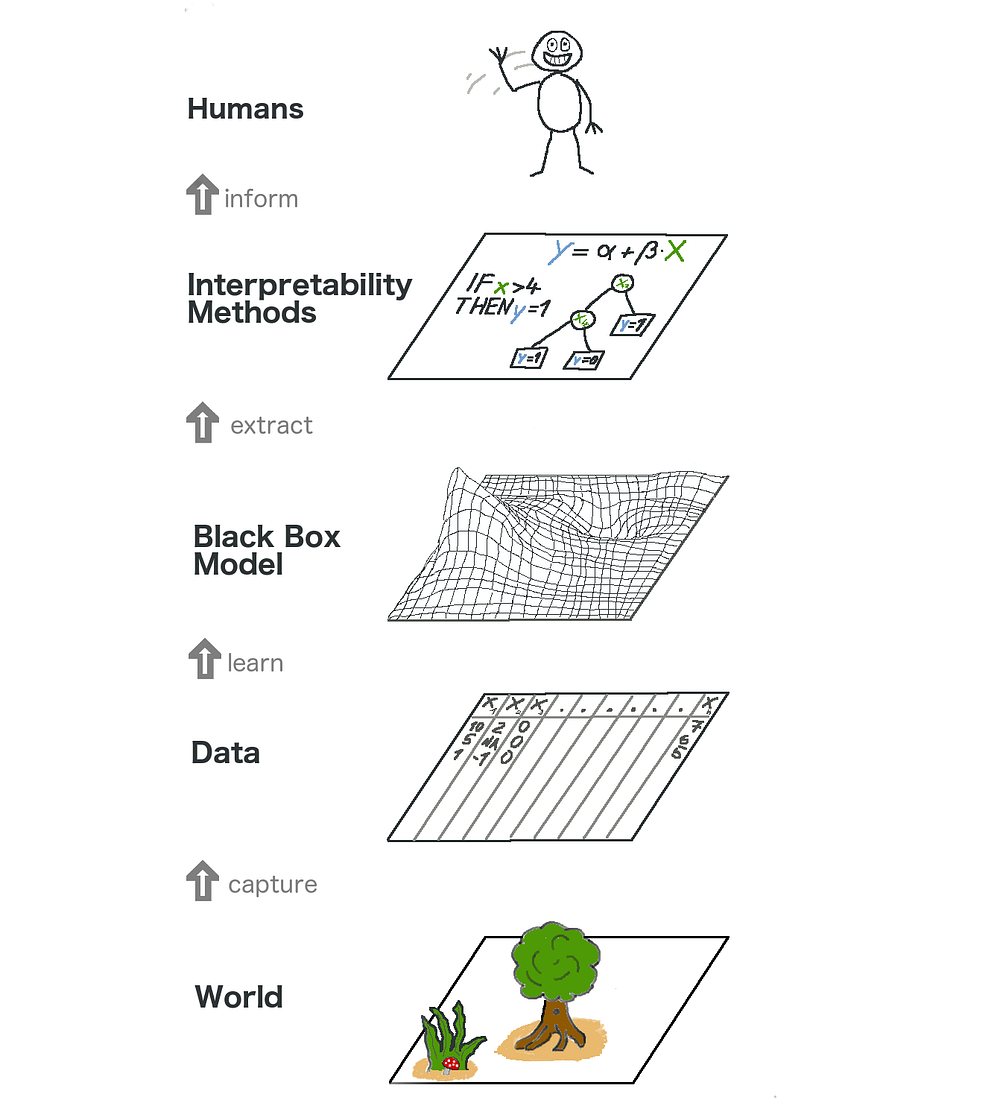Some of the benefits that interpretability brings along are:

• Reliability
• Debugging
• Informing feature engineering
• Directing future data collection
• Informing human decision-making
• Building Trust

## Model Explainability techniques

Theory only makes sense as long as we can put it into practice. In case you want a real hang of this topic, you can try the Machine Learning Explainability crash course from Kaggle. It has the right amount of theory and code to put the concepts into perspective and helps to apply model explainability concepts to practical, real-world problems.

### Insights which can be extracted from the models

To interpret a model, we require the following insights :

• Features in the model which are most important.
• For any single prediction from a model, the effect of each feature in the data on that particular prediction.
• Effect of each feature over a large number of possible predictions

Let’s discuss a few techniques which help in extracting the above insights from a model:

### 1. Permutation Importance

What features does a model think are important? Which features might have a greater impact on the model predictions than the others? This concept is called feature importance and Permutation Importance is a technique used widely for calculating feature importance. It helps us to see when our model produces counterintuitive results, and it helps to show the others when our model is working as we’d hope.

Permutation Importance works for many scikit-learn estimators. The idea is simple: Randomly permutate or shuffle a single column in the validation dataset leaving all the other columns intact. A feature is considered “important” if the model’s accuracy drops a lot and causes an increase in error. On the other hand, a feature is considered ‘unimportant’ if shuffling its values don’t affect the model’s accuracy.

Working

Consider a model that predicts whether a soccer team will have a “Man of the Game” winner or not based on certain parameters. The player who demonstrates the best play is awarded this title.

Permutation importance is calculated after a model has been fitted. So, let’s train and fit a RandomForestClassifier model denoted as my_model on the training data.

Permutation Importance is calculated using the ELI5 library. ELI5 is a Python library which allows to visualize and debug various Machine Learning models using unified API. It has built-in support for several ML frameworks and provides a way to explain black-box models.

Calculating and Displaying importance using the eli5 library:

(Here `val_X,val_y` denote the validation sets respectively)

``````import eli5
from eli5.sklearn import PermutationImportance

perm = PermutationImportance(my_model, random_state=1).fit(val_X, val_y)
eli5.show_weights(perm, feature_names = val_X.columns.tolist())``````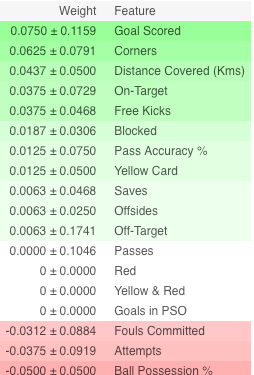Interpretation

• The features at the top are most important and at the bottom, the least. For this example, goals scored was the most important feature.
• The number after the ± measures how performance varied from one-reshuffling to the next.
• Some weights are negative. This is because in those cases predictions on the shuffled data were found to be more accurate than the real data.

Practice

And now, for the complete example and to test your understanding, go to the Kaggle page by clicking the link below:

Get Started Here

### 2. Partial Dependence Plots

The partial dependence plot (short PDP or PD plot) shows the marginal effect one or two features have on the predicted outcome of a machine learning model( J. H. Friedman 2001). PDPs show how a feature affects predictions. PDP can show the relationship between the target and the selected features via 1D or 2D plots.

Working

PDPs are also calculated after a model has been fit. In the soccer problem that we discussed above, there were a lot of features like passes made, shots taken, goals scored etc. We start by considering a single row. Say the row represents a team that had the ball 50% of the time, made 100 passes, took 10 shots and scored 1 goal.

We proceed by fitting our model and calculating the probability of a team having a player that won the “Man of the Game” which is our target variable. Next, we would choose a variable and continuously alter its value. For instance, we will calculate the outcome if the team scored 1 goal, 2 goals, 3 goals and so on. All these values are then plotted and we get a graph of predicted Outcomes vs Goals Scored.

The library to be used for plotting PDPs is called python partial dependence plot toolbox or simply PDPbox.

``````from matplotlib import pyplot as plt
from pdpbox import pdp, get_dataset, info_plots

# Create the data that we will plot
pdp_goals = pdp.pdp_isolate(model=my_model, dataset=val_X, model_features=feature_names, feature='Goal Scored')

# plot it
pdp.pdp_plot(pdp_goals, 'Goal Scored')
plt.show()``````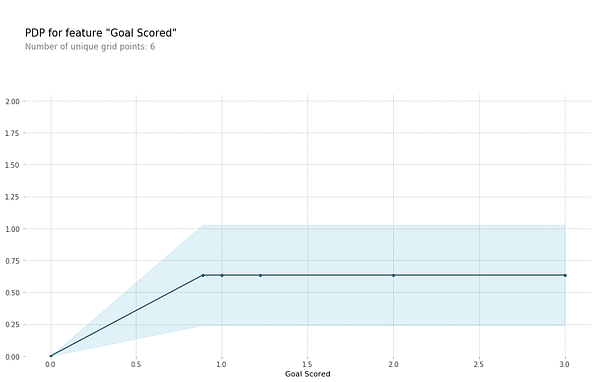Interpretation

• The Y-axis represents the change in prediction from what it would be predicted at the baseline or leftmost value.
• Blue area denotes the confidence interval
• For the ‘Goal Scored’ graph, we observe that scoring a goal increases the probability of getting a ‘Man of the game’ award but after a while saturation sets in.

We can also visualize the partial dependence of two features at once using 2D Partial plots.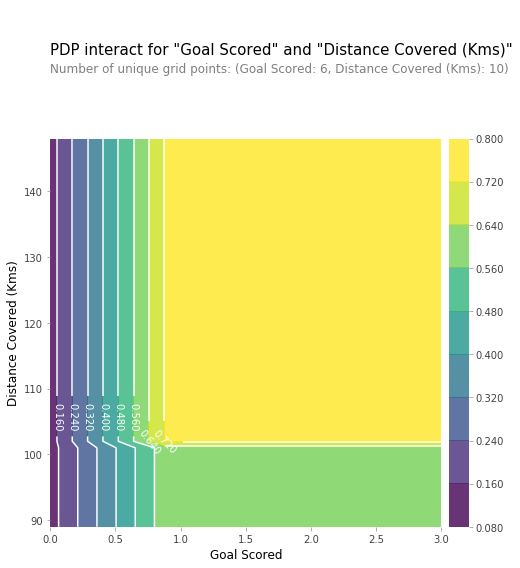Practise Get Started Here

### 3. SHAP Values

SHAP which stands for SHapley Additive exPlanation, helps to break down a prediction to show the impact of each feature. It is based on Shapley values, a technique used in game theory to determine how much each player in a collaborative game has contributed to its success¹. Normally, getting the trade-off between accuracy and interpretability just right can be a difficult balancing act but SHAP values can deliver both.

Working

Again, going with the soccer example where we wanted to predict the probability of a team having a player that won the “Man of the Game”. SHAP values interpret the impact of having a certain value for a given feature in comparison to the prediction we’d make if that feature took some baseline value.

SHAP values are calculated using the Shap library which can be installed easily from PyPI or conda.

Shap values show how much a given feature changed our prediction (compared to if we made that prediction at some baseline value of that feature). Let’s say we wanted to know what was the prediction when the team scored 3 goals instead of some fixed baseline no. If we are able to answer this, we could perform the same steps for other features as follows:

``sum(SHAP values for all features) = pred_for_team - pred_for_baseline_values``

Hence the prediction can be decomposed into a graph like this: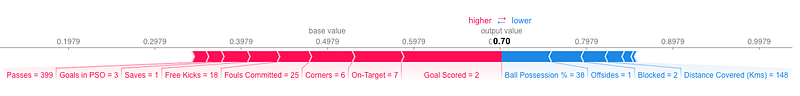Interpretation

The above explanation shows features each contributing to push the model output from the base value (the average model output over the training dataset we passed) to the model output. Features pushing the prediction higher are shown in red, those pushing the prediction lower are in blue

• The base_value here is 0.4979 while our predicted value is 0.7.
• `Goal Scored` = 2 has the biggest impact on increasing the prediction, while
• `ball possession` feature has the biggest effect in decreasing the prediction.

Practice

SHAP values have a deeper theory than what I have explained here. make sure to through the link below to get a complete understanding. Get Started Here

### 4. Advanced Uses of SHAP Values

Aggregating many SHAP values can provide even more detailed insights into the model.

• SHAP Summary Plots

To get an overview of which features are most important for a model we can plot the SHAP values of every feature for every sample. The summary plot tells which features are most important, and also their range of effects over the dataset.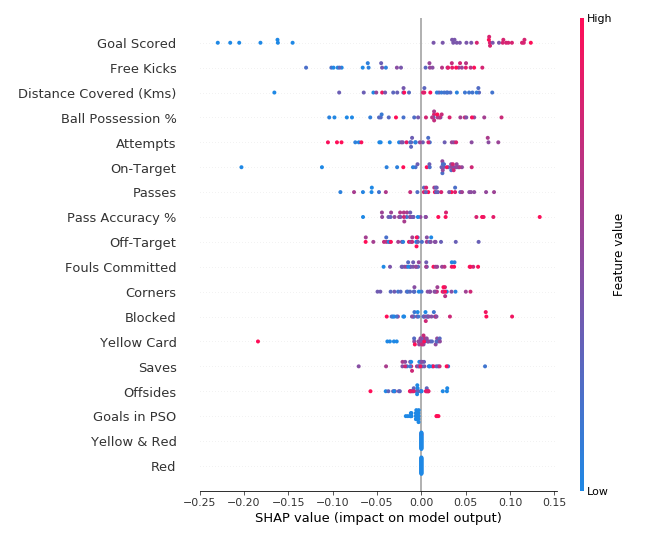For every dot:

• Vertical location shows what feature it is depicting
• Color shows whether that feature was high or low for that row of the dataset
• Horizontal location shows whether the effect of that value caused a higher or lower prediction.

The point in the upper left was for a team that scored few goals, reducing the prediction by 0.25.

• SHAP Dependence Contribution Plots

While a SHAP summary plot gives a general overview of each feature, a SHAP dependence plot shows how the model output varies by a feature value. SHAP dependence contribution plots provide a similar insight to PDP’s, but they add a lot more detail.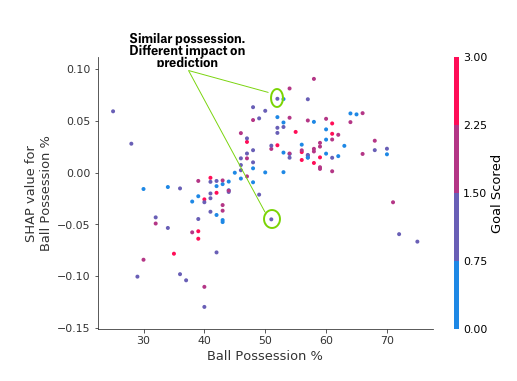The above Dependence Contribution plots suggest that having the ball increases a team’s chance of having their player win the award. But if they only score one goal, that trend reverses and the award judges may penalize them for having the ball so much if they score that little.

Get Started Here

## Conclusion

Machine Learning doesn’t have to be a black box anymore. What use is a good model if we cannot explain the results to others. Interpretability is as important as creating a model. To achieve wider acceptance among the population, it is crucial that Machine learning systems are able to provide satisfactory explanations for their decisions. As Albert Einstein said,” If you can’t explain it simply, you don’t understand it well enough”.

## Intro to Data Science - Crash Course for Beginners

Learn the basic components of Data Science in this crash course for beginners. You will learn: Statistics; Data visualization; Programming

## Python Tutorial - Python GUI Programming - Python GUI Examples (Tkinter Tutorial)

In this tutorial, we will learn how to develop graphical user interfaces by writing some Python GUI examples using the Tkinter package.

## Learn Data Science Tutorial - Full Course for Beginners

Learn Data Science is this full tutorial course for absolute beginners. Data science is considered the "sexiest job of the 21st century." You'll learn the important elements of data science. You'll be introduced to the principles, practices, and tools that make data science the powerful medium for critical insight in business and research. You'll have a solid foundation for future learning and applications in your work. With data science, you can do what you want to do, and do it better. This course covers the foundations of data science, data sourcing, coding, mathematics, and statistics.

## 5 Python Online Courses for Beginners

If you are thinking to learn a new programming language then also Python is a good choice, particularly if you are looking to move towards a lucrative career path of Data Science and Machine learning which has lots of opportunities. In this article, I am going to share some of the best online courses to learn Python in 2020...

## What NOT to Do in the Data Science Domains Industry

Data science is linked to numerous other modern buzzwords such as big data and machine learning, but data science itself is built from numerous domains, where you can get your expertise. These domains include the following: * Statistics *...